# A Level Statistics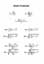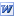Statistics Formula (Part 1) Statistics 1 formula covering the single maths Stats topics. Notes for the older AQA syllabus (PDF here)and the OCR syllabus (PDF here).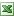Central Limit Theorem Graphic demonstration of the Central Limit Theorem. Use sliders to change shapes of graphs. Two tabs - one to demonstrate non-normal distributions, one to demonstrate standard deviation of estimations of normal distributions.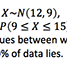Statistics Distributions Summary document highlighting key features, criteria, examples, notation and equations of the binomial, normal, poisson and geometric distributions. PDF here.Graphs of Distributions Dynamic graphs of the binomial, normal and poisson distributions. Use sliders to change values and shapes.Product Moment Correlation Coefficient Scattergraph vs PMCC vs sentence, in ascending order.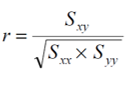Correlation and Regression Formulae The formulae for calculating a, b, r and lines of best, correlation values. PDF here.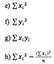Least Squares Regression Lines In this task students begin with recapping scatter diagrams before continuing on to find the equation of a least squares regression line. The intention being to make the link as explicit and direct as possible. Answers included. Pdf version.Hypothesis Testing Terminology Notes and examples of common hypothesis testing terminology. PDF here.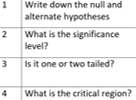Notes on the Hypothesis Testing Proceedure Notes on the standard hypothesis testing proceedure. Break the question down into smaller chunks by writing these key steps down.PDF here. More notes here, including testing for correlation and confidence intervals. PDF here.Hypothesis Testing Exam Questions Three exam questions, and then three more, together with a writing frame to emphasise the similar structure in hypothesis testing questions between the proceedure of hypothesis testing with binomial, normal and product moment. Includes answers. Pdf version.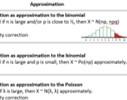Distribution Approximations Notes on using stats distributions as approximations to other stats distributions . Pdf version.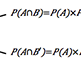Independent Vs Non-Independent Two tree diagrams to illustrate the difference between probabilities of independent events and non-independent events. PDF here.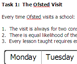Probability Ofsted Pupils use logic and combinations to find the probability of extra planning for lessons when Ofsted visits a school. Not easy - most able students only - and definately an open-ended task that Ofsted would enjoy should they visit the lesson!Zip file of 4 exam papers plus an Excel file (answer grid) detailing each type of question. Good activity for students familiarise themselves with recognising the types of question. Print out the exam papers (on different coloured card), cut up each question and ask pupils to match up all the binomials, geomatrics, perms & combs, prob dists, Spearmans ranks etc.Cakes & Celebrities A great starter to introduce Spearmans Rank Correlation Coefficient. Print out two sets of cards, cut up and ask two pupils to order them according to preference. Compare pupils orders using Spearmans Rank. For the celebrities suggest 'who would they most prefer to go to dinner with'.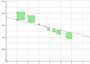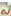Autograph File: Linear Regression A nice starter example and then the main activity is four sets of data for pupils to plot scatter diagrams and discover if positive, negative, weak strong or no correlation. This is a great activity if used on Autograph as pupils can then add a least squares regression line and find the equation of etc. Completed Autograph files of each set are here: 1, 2, 3, 4. Or everything as a is zip file.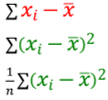Calculating Standard Deviation A good explanation and introduction to standard deviation, what it is and how to calculate it. PDF here.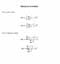Variance Formula Variance formula, typed out neatly so you don't have to write it!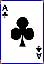To download images of a pack of cards as a single zip file.